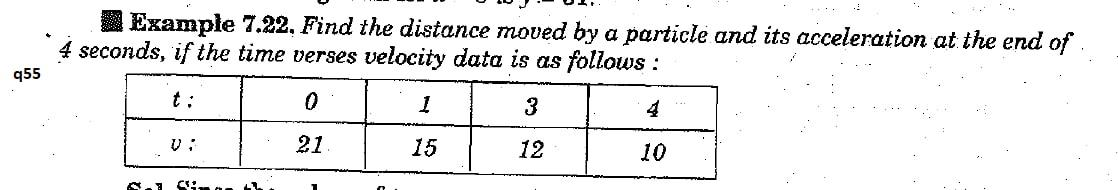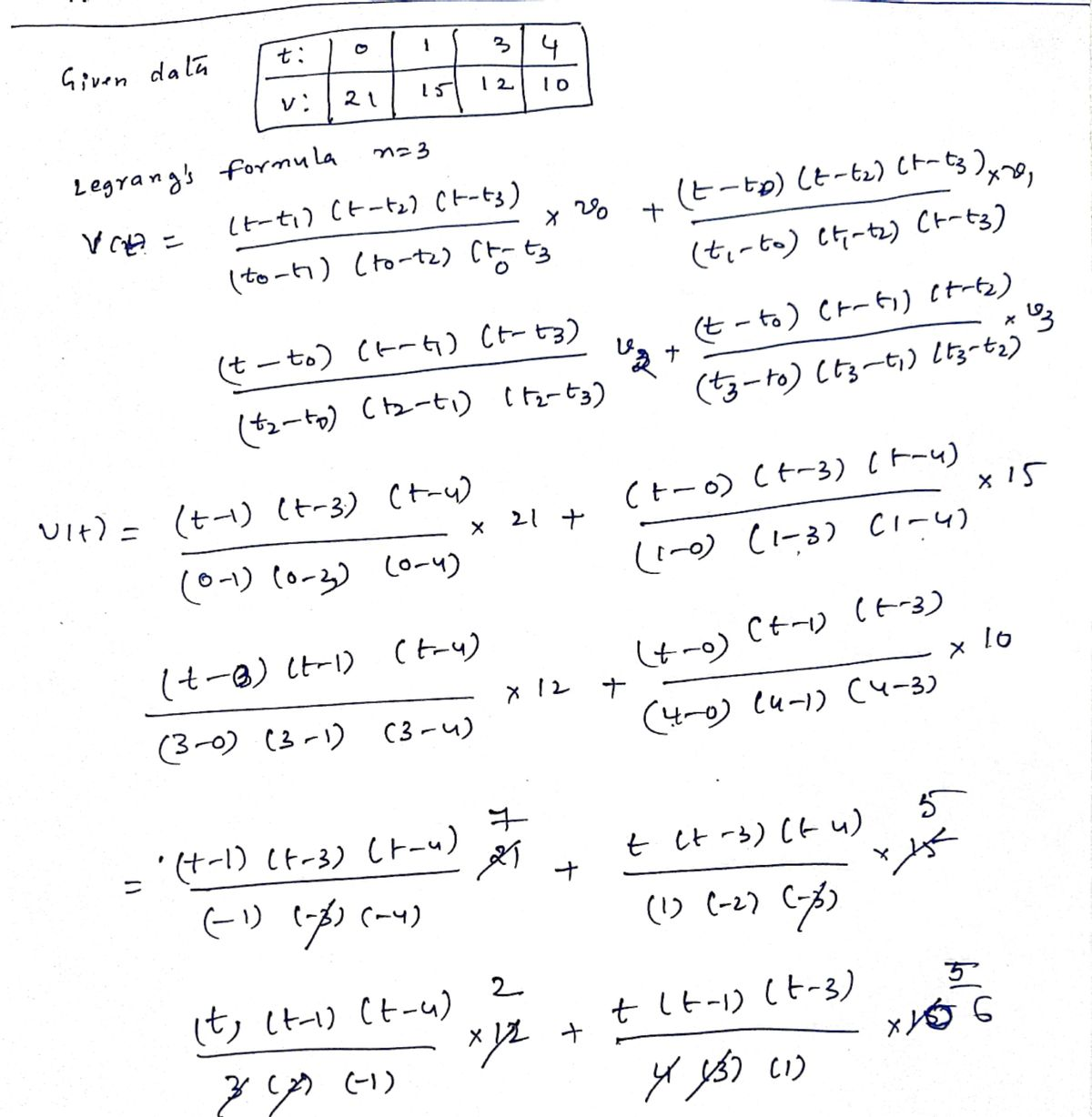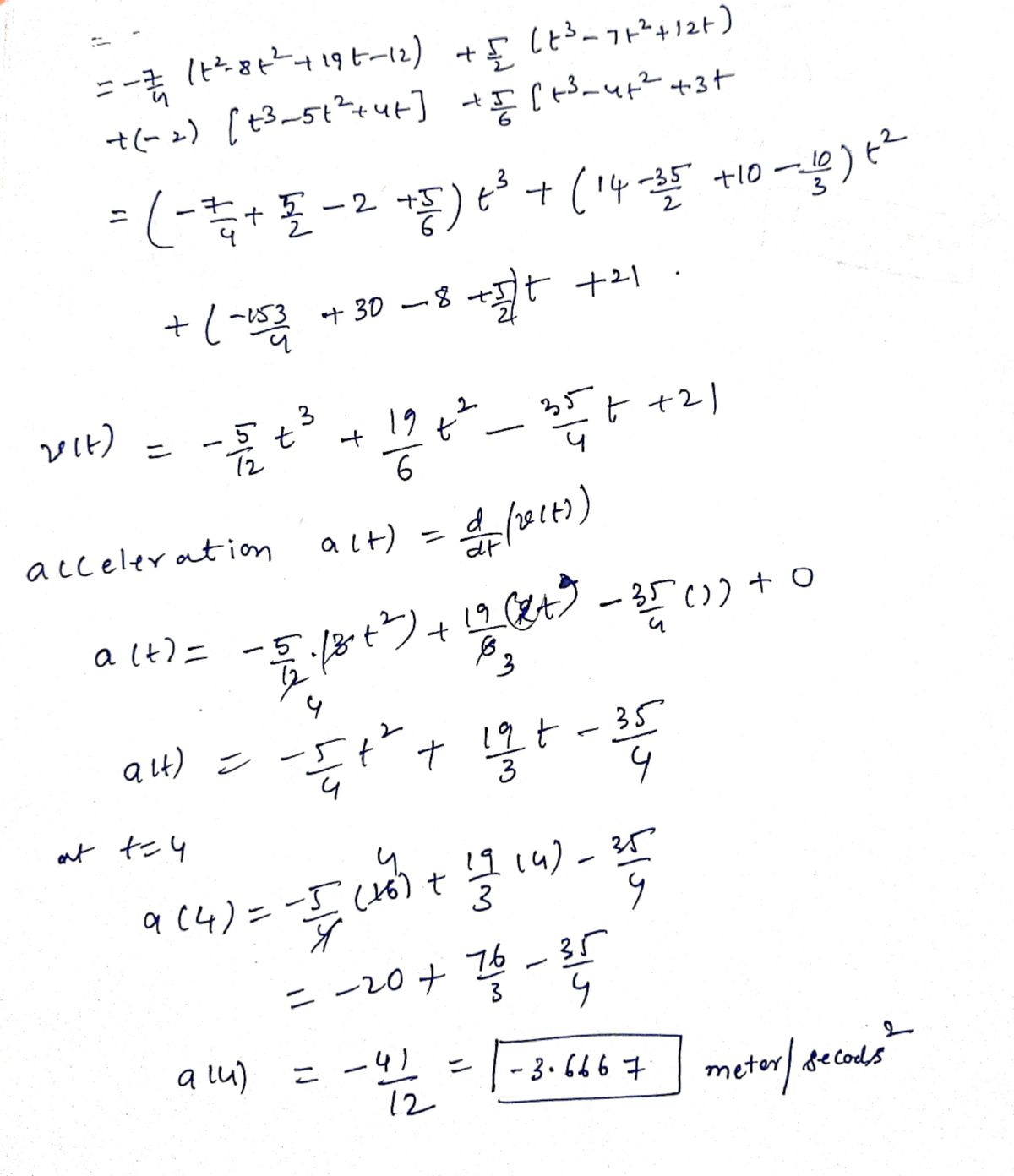# Example 7.22, Find the distance moved by a particle and its acceleration at the end of 4 seconds, if the time verses velocity Example 7.22, Find the distance moved by a particle and its acceleration at the end of 4 seconds, if the time verses velocity data is as follows

Question-AnswerCategory: MathematicsExample 7.22, Find the distance moved by a particle and its acceleration at the end of 4 seconds, if the time verses velocity Example 7.22, Find the distance moved by a particle and its acceleration at the end of 4 seconds, if the time verses velocity data is as followsExample 7.22, Find the distance moved by a particle and its acceleration at the end of 4 seconds, if the time verses velocity data is as follows